# Mu0Poly Class Reference [Species Reference-State Thermodynamic Properties]

The Mu0Poly class implements an interpolation of the Gibbs free energy based on a piecewise constant heat capacity approximation. More...

#include <Mu0Poly.h>

Inheritance diagram for Mu0Poly: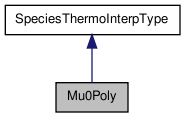[legend]
Collaboration diagram for Mu0Poly:[legend]

List of all members.

## Public Member Functions

Mu0Poly ()
Constructor.
Mu0Poly (int n, doublereal tlow, doublereal thigh, doublereal pref, const doublereal *coeffs)
Constructor used in templated instantiations.
Mu0Poly (const Mu0Poly &)
Copy constructor.
Mu0Polyoperator= (const Mu0Poly &)
Assignment operator.
virtual ~Mu0Poly ()
Destructor.
virtual SpeciesThermoInterpTypeduplMyselfAsSpeciesThermoInterpType () const
Duplicator.
virtual doublereal minTemp () const
Returns the minimum temperature that the thermo parameterization is valid.
virtual doublereal maxTemp () const
Returns the maximum temperature that the thermo parameterization is valid.
virtual doublereal refPressure () const
Returns the reference pressure (Pa).
virtual int reportType () const
Returns an integer representing the type of parameterization.
virtual int speciesIndex () const
Returns an integer representing the species index.
virtual void updateProperties (const doublereal *tPoly, doublereal *cp_R, doublereal *h_RT, doublereal *s_R) const
Update the properties for this species, given a temperature polynomial.
virtual void updatePropertiesTemp (const doublereal temp, doublereal *cp_R, doublereal *h_RT, doublereal *s_R) const
Compute the reference-state property of one species.
virtual void reportParameters (int &n, int &type, doublereal &tlow, doublereal &thigh, doublereal &pref, doublereal *const coeffs) const
This utility function reports back the type of parameterization and all of the parameters for the species, index.
virtual void modifyParameters (doublereal *coeffs)
Modify parameters for the standard state.

## Protected Attributes

int m_numIntervals
Number of intervals in the interpolating linear approximation.
doublereal m_H298
Value of the enthalpy at T = 298.15.
vector_fp m_t0_int
Points at which the standard state chemical potential are given.
vector_fp m_mu0_R_int
Mu0's are primary input data.
vector_fp m_h0_R_int
Dimensionless Enthalpies at the temperature points.
vector_fp m_s0_R_int
Entropy at the points.
vector_fp m_cp0_R_int
Heat capacity at the points.
doublereal m_lowT
Limiting low temperature.
doublereal m_highT
Limiting high temperature.
doublereal m_Pref
Reference pressure.
int m_index
Species index.

## Private Member Functions

void processCoeffs (const doublereal *coeffs)
process the coefficients

## Detailed Description

The Mu0Poly class implements an interpolation of the Gibbs free energy based on a piecewise constant heat capacity approximation.

The Mu0Poly class implements a piecewise constant heat capacity approximation. of the standard state chemical potential of one species at a single reference pressure. The chemical potential is input as a series of (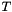,) values. The first temperature is assumed to be equal to 298.15 K; however, this may be relaxed in the future. This information, and an assumption of a constant heat capacity within each interval is enough to calculate all thermodynamic functions.

The piece-wise constant heat capacity is calculated from the change in the chemical potential over each interval. Once the heat capacity is known, the other thermodynamic functions may be determined. The basic equation for going from temperature point 1 to temperature point 2 are as follows for,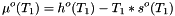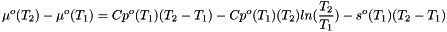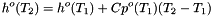Within each interval the following relations are used. For,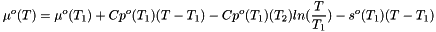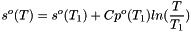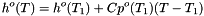Notes about temperature interpolation for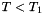and. These are achieved by assuming a constant heat capacity equal to the value in the closest temperature interval. No error is thrown.

Note:
In the future, a better assumption about the heat capacity may be employed, so that it can be continuous.

Definition at line 78 of file Mu0Poly.h.

## Constructor & Destructor Documentation

 Mu0Poly ( )

Constructor.

Definition at line 28 of file Mu0Poly.cpp.

Referenced by Mu0Poly::duplMyselfAsSpeciesThermoInterpType().

 Mu0Poly ( int n, doublereal tlow, doublereal thigh, doublereal pref, const doublereal * coeffs )

Constructor used in templated instantiations.

In the constructor, we calculate and store the piecewise linear approximation to the thermodynamic functions.

Parameters:
 n Species index tlow Minimum temperature thigh Maximum temperature pref reference pressure (Pa). coeffs Vector of coefficients used to set the parameters for the standard state for species n. There are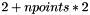coefficients, where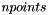are the number of temperature points. Their identity is further broken down: coeffs = number of points (integer) coeffs =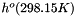(J/kmol) coeffs =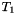(Kelvin) coeffs =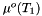(J/kmol) coeffs =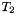(Kelvin) coeffs =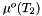(J/kmol) coeffs =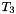(Kelvin) coeffs =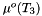(J/kmol) ........

Definition at line 53 of file Mu0Poly.cpp.

References Mu0Poly::processCoeffs().

 Mu0Poly ( const Mu0Poly & b )

Copy constructor.

Definition at line 67 of file Mu0Poly.cpp.

 ~Mu0Poly ( )  [virtual]

Destructor.

Definition at line 101 of file Mu0Poly.cpp.

## Member Function Documentation

 SpeciesThermoInterpType * duplMyselfAsSpeciesThermoInterpType ( ) const [virtual]

Duplicator.

Implements SpeciesThermoInterpType.

Definition at line 105 of file Mu0Poly.cpp.

References Mu0Poly::Mu0Poly().

 doublereal maxTemp ( ) const [virtual]

Returns the maximum temperature that the thermo parameterization is valid.

Implements SpeciesThermoInterpType.

Definition at line 111 of file Mu0Poly.cpp.

References Mu0Poly::m_highT.

 doublereal minTemp ( ) const [virtual]

Returns the minimum temperature that the thermo parameterization is valid.

Implements SpeciesThermoInterpType.

Definition at line 110 of file Mu0Poly.cpp.

References Mu0Poly::m_lowT.

 void modifyParameters ( doublereal * coeffs )  [virtual]

Modify parameters for the standard state.

Parameters:
 coeffs Vector of coefficients used to set the parameters for the standard state.

Reimplemented from SpeciesThermoInterpType.

Definition at line 182 of file Mu0Poly.cpp.

References Mu0Poly::processCoeffs().

 Mu0Poly & operator= ( const Mu0Poly & b )

Assignment operator.

Definition at line 81 of file Mu0Poly.cpp.

 void processCoeffs ( const doublereal * coeffs )  [private]

process the coefficients

In the constructor, we calculate and store the piecewise linear approximation to the thermodynamic functions.

Parameters:
 coeffs coefficients. These are defined as follows:

coeffs = number of points (integer) 1 = H298(J/kmol) 2 = T1 (Kelvin) 3 = mu1 (J/kmol) 4 = T2 (Kelvin) 5 = mu2 (J/kmol) 6 = T3 (Kelvin) 7 = mu3 (J/kmol) ........

Definition at line 293 of file Mu0Poly.cpp.

Referenced by Mu0Poly::modifyParameters(), and Mu0Poly::Mu0Poly().

 doublereal refPressure ( ) const [virtual]

Returns the reference pressure (Pa).

Implements SpeciesThermoInterpType.

Definition at line 112 of file Mu0Poly.cpp.

References Mu0Poly::m_Pref.

 void reportParameters ( int & n, int & type, doublereal & tlow, doublereal & thigh, doublereal & pref, doublereal *const coeffs ) const [virtual]

This utility function reports back the type of parameterization and all of the parameters for the species, index.

All parameters are output variables

Parameters:
 n Species index type Integer type of the standard type tlow output - Minimum temperature thigh output - Maximum temperature pref output - reference pressure (Pa). coeffs Vector of coefficients used to set the parameters for the standard state.

Implements SpeciesThermoInterpType.

Definition at line 163 of file Mu0Poly.cpp.

 virtual int reportType ( ) const [inline, virtual]

Returns an integer representing the type of parameterization.

Implements SpeciesThermoInterpType.

Definition at line 140 of file Mu0Poly.h.

References MU0_INTERP.

 virtual int speciesIndex ( ) const [inline, virtual]

Returns an integer representing the species index.

Implements SpeciesThermoInterpType.

Definition at line 143 of file Mu0Poly.h.

References Mu0Poly::m_index.

 void updateProperties ( const doublereal * tPoly, doublereal * cp_R, doublereal * h_RT, doublereal * s_R ) const [virtual]

Update the properties for this species, given a temperature polynomial.

This method is called with a pointer to an array containing the functions of temperature needed by this parameterization, and three pointers to arrays where the computed property values should be written. This method updates only one value in each array.

Temperature Polynomial:

tPoly = temp (Kelvin)

Parameters:
 tPoly vector of temperature polynomials. Length = 1 cp_R Vector of Dimensionless heat capacities. (length m_kk). h_RT Vector of Dimensionless enthalpies. (length m_kk). s_R Vector of Dimensionless entropies. (length m_kk).

Reimplemented from SpeciesThermoInterpType.

Definition at line 129 of file Mu0Poly.cpp.

Referenced by Mu0Poly::updatePropertiesTemp().

 void updatePropertiesTemp ( const doublereal temp, doublereal * cp_R, doublereal * h_RT, doublereal * s_R ) const [virtual]

Compute the reference-state property of one species.

Given temperature T in K, this method updates the values of the non-dimensional heat capacity at constant pressure, enthalpy, and entropy, at the reference pressure, Pref of one of the species. The species index is used to reference into the cp_R, h_RT, and s_R arrays.

Parameters:
 temp Temperature (Kelvin) cp_R Vector of Dimensionless heat capacities. (length m_kk). h_RT Vector of Dimensionless enthalpies. (length m_kk). s_R Vector of Dimensionless entropies. (length m_kk).

Implements SpeciesThermoInterpType.

Definition at line 150 of file Mu0Poly.cpp.

References Mu0Poly::updateProperties().

## Member Data Documentation

 vector_fp m_cp0_R_int [protected]

Heat capacity at the points.

Definition at line 250 of file Mu0Poly.h.

Referenced by Mu0Poly::operator=(), Mu0Poly::processCoeffs(), and Mu0Poly::updateProperties().

 vector_fp m_h0_R_int [protected]

Dimensionless Enthalpies at the temperature points.

Definition at line 244 of file Mu0Poly.h.

Referenced by Mu0Poly::operator=(), Mu0Poly::processCoeffs(), and Mu0Poly::updateProperties().

 doublereal m_H298 [protected]

Value of the enthalpy at T = 298.15.

This value is tied to the Heat of formation of the species at 298.15.

Definition at line 229 of file Mu0Poly.h.

Referenced by Mu0Poly::operator=(), Mu0Poly::processCoeffs(), and Mu0Poly::reportParameters().

 doublereal m_highT [protected]

Limiting high temperature.

Definition at line 254 of file Mu0Poly.h.

Referenced by Mu0Poly::maxTemp(), Mu0Poly::operator=(), and Mu0Poly::reportParameters().

 int m_index [protected]

Species index.

Definition at line 260 of file Mu0Poly.h.

 doublereal m_lowT [protected]

Limiting low temperature.

Definition at line 252 of file Mu0Poly.h.

Referenced by Mu0Poly::minTemp(), Mu0Poly::operator=(), and Mu0Poly::reportParameters().

 vector_fp m_mu0_R_int [protected]

Mu0's are primary input data.

They aren't strictly needed, but are kept here for convenience.

Definition at line 241 of file Mu0Poly.h.

Referenced by Mu0Poly::operator=(), Mu0Poly::processCoeffs(), and Mu0Poly::reportParameters().

 int m_numIntervals [protected]

Number of intervals in the interpolating linear approximation.

Number of points is one more than the number of intervals.

Definition at line 222 of file Mu0Poly.h.

 doublereal m_Pref [protected]

Reference pressure.

Definition at line 257 of file Mu0Poly.h.

Referenced by Mu0Poly::operator=(), Mu0Poly::refPressure(), and Mu0Poly::reportParameters().

 vector_fp m_s0_R_int [protected]

Entropy at the points.

Definition at line 247 of file Mu0Poly.h.

Referenced by Mu0Poly::operator=(), Mu0Poly::processCoeffs(), and Mu0Poly::updateProperties().

 vector_fp m_t0_int [protected]

Points at which the standard state chemical potential are given.

Definition at line 235 of file Mu0Poly.h.

The documentation for this class was generated from the following files:
Generated by1.6.3